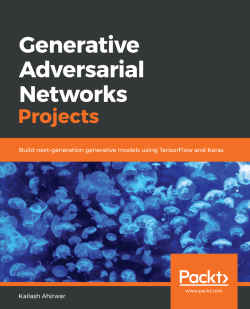By Kailash Ahirwar
• Constantly updated with 100+ new titles each month
• Breadth and depth in over 1,000+ technologies

Generative Adversarial Networks (GANs) have the potential to build next-generation models, as they can mimic any distribution of data. Major research and development work is being undertaken in this field since it is one of the rapidly growing areas of machine learning. This book will test unsupervised techniques for training neural networks as you build seven end-to-end projects in the GAN domain.

Generative Adversarial Network Projects begins by covering the concepts, tools, and libraries that you will use to build efficient projects. You will also use a variety of datasets for the different projects covered in the book. The level of complexity of the operations required increases with every chapter, helping you get to grips with using GANs. You will cover popular approaches such as 3D-GAN, DCGAN, StackGAN, and CycleGAN, and you’ll gain an understanding of the architecture and functioning of generative models through their practical implementation.

By the end of this book, you will be ready to build, train, and optimize your own end-to-end GAN models at work or in your own projects.

Publication date:
January 2019
Publisher
Packt
Pages
316
ISBN
9781789136678

## ChapterÂ 1.Â Introduction to Generative Adversarial Networks

In this chapter, we will look at Generative Adversarial Networks (GANs). They are a type of deep neural network architecture that uses unsupervised machine learning to generate data. TheyÂ were introducedÂ in 2014,Â in a paper by Ian Goodfellow, Yoshua Bengio, and Aaron Courville, which can be found at the following link:Â https://arxiv.org/pdf/1406.2661.Â GANs have many applications, including image generation and drug development.

This chapter will introduce you to the core components of GANs. It will take you through how each component works and the important concepts and technology behind GANs. It will also give you a brief overview of the benefits and drawbacksÂ of using GANs and an insight into certain real-world applications.

The chapter will cover all of these points by exploring the following topics:

• What is a GAN?
• The architecture of a GAN
• Important concepts related to GANs
• Different varieties of GANs
• Practical applications of GANs

## What is a GAN?

A GAN is a deep neural network architecture made up of two networks, a generator network and a discriminator network. Through multiple cycles of generation and discrimination, both networks train each other, while simultaneously trying to outwit each other.

### What is a generator network?

A generator network uses existing data to generate new data. It can, for example, use existing images to generate new images.Â TheÂ generator's primary goal is to generate data (such as images, video, audio, or text) from a randomly generated vector of numbers, called a latent space. While creating a generator network, we need to specify the goal of the network. This might be image generation, text generation, audio generation, video generation, and so on.

### What is a discriminator network?

The discriminator network tries to differentiate between the real data and the data generated by the generator network. The discriminator network tries to put the incoming data into predefined categories. It can either perform multi-class classification or binary classification. Generally, in GANs binary classification is performed.

### Training through adversarial play in GANs

In a GAN, the networks are trained through adversarial play: both networks compete against each other. As an example, let's assume that we want theÂ GAN to create forgeries of artworks:

1. The first network, the generator, has never seen the real artwork but is trying to create an artwork that looks like the real thing.
2. The second network, the discriminator, tries to identify whether an artwork is real or fake.
3. The generator, in turn, tries to fool the discriminator into thinking that its fakes are the real deal by creating more realistic artwork over multiple iterations.
4. The discriminator tries to outwit the generator by continuing to refine its own criteria for determining a fake.
5. They guide each other by providing feedback from the successful changes they make in their own process in each iteration. This process is the training of the GAN.
6. Ultimately, the discriminator trains the generator to the point at which it can no longer determine which artwork is real and which is fake.

In this game, both networks are trained simultaneously. When we reach a stage at which the discriminator is unable to distinguish between real and fake artworks, the network attains a state known as Nash equilibrium. This will be discussed later on in this chapter.

## Practical applications of GANs

GANs have some fairly useful practical applications, which include the following:

• Image generation:Â Generative networks can be used to generate realistic images after being trained on sample images. For example, if we want to generate new images of dogs, we can train a GAN on thousands of samples ofÂ images of dogs. Once the training has finished, the generator network will be able to generate new images that are different from the images in the training set. Image generation is used in marketing, logo generation, entertainment, social media, and so on. In the next chapter, we will be generating faces of anime characters.
• Text-to-image synthesis:Â Generating images from text descriptions is an interesting use case of GANs. This can be helpful in the film industry, as a GAN is capable of generating new data based on some text that you have made up. In the comic industry, it is possible to automatically generate sequences of a story.
• Face aging:Â This can be very useful for both the entertainment and surveillance industries. It is particularly useful for face verification because it means that aÂ company doesn't need to change their security systems asÂ people get older. An age-cGAN network can generate images at different ages, which can then be used to train a robust model for face verification.
• Image-to-image translation:Â Image-to-image translation can be used to convert images taken in the day to images taken at night, to convert sketches to paintings, to style images to look like Picasso or Van Gogh paintings, to convert aerial images to satellite imagesÂ automatically, and toÂ convert images of horses to images of zebras. These use cases are ground-breaking because they can save us time.
• Video synthesis:Â GANs can also be used to generate videos. They can generate content in less time than if we were to create content manually. They can enhance the productivity of movie creators and also empower hobbyists who want to make creative videos in their free time.
• High-resolution image generation:Â If you have pictures taken from a low-resolution camera, GANs can help you generate high-resolution images without losing any essential details. This can be useful on websites.
• Completing missing parts of images:Â If you have an image that has some missing parts, GANs can help you to recover these sections.

## The detailed architecture of a GAN

The architecture of a GAN has two basic elements: the generator network and the discriminator network. Each network can be any neural network, such as an Artificial Neural NetworkÂ (ANN), aÂ Convolutional Neural NetworkÂ (CNN), aÂ Recurrent Neural NetworkÂ (RNN), or a Long Short Term MemoryÂ (LSTM). The discriminator has to have fully connected layers with a classifier at the end.

Let's take a closer look at the components of the architecture of a GAN. In this example, we will imagine that we are creating a dummy GAN.

### The architecture of the generatorÂ

The generator network in our dummy GAN is a simple feed-forward neural network with five layers: an input layer, three hidden layers, and an output layer. Let's take a closer look at the configuration of the generator (dummy)Â network:

 Layer # Layer name Configuration 1 Input layer `input_shape=(batch_size, 100)`, `output_shape=(batch_size, 100)` 2 Dense layer `neurons=500`, `input_shape=(batch_size, 100)`,`output_shape=(batch_size, 500)` 3 Dense layer `neurons=500`, `input_shape=(batch_size, 500)`,`output_shape=(batch_size, 500)` 4 Dense layer `neurons=784`, `input_shape=(batch_size, 500)`, `output_shape=(batch_size, 784)` 5 Reshape layer `input_shape=(batch_size, 784)`, `output_shape=(batch_size, 28, 28)`

Â

The preceding table shows the configurations of the hidden layers, and also the input and output layers in the network.

The following diagram shows the flow of tensors and the input and output shapes of the tensors for each layer in the generator network: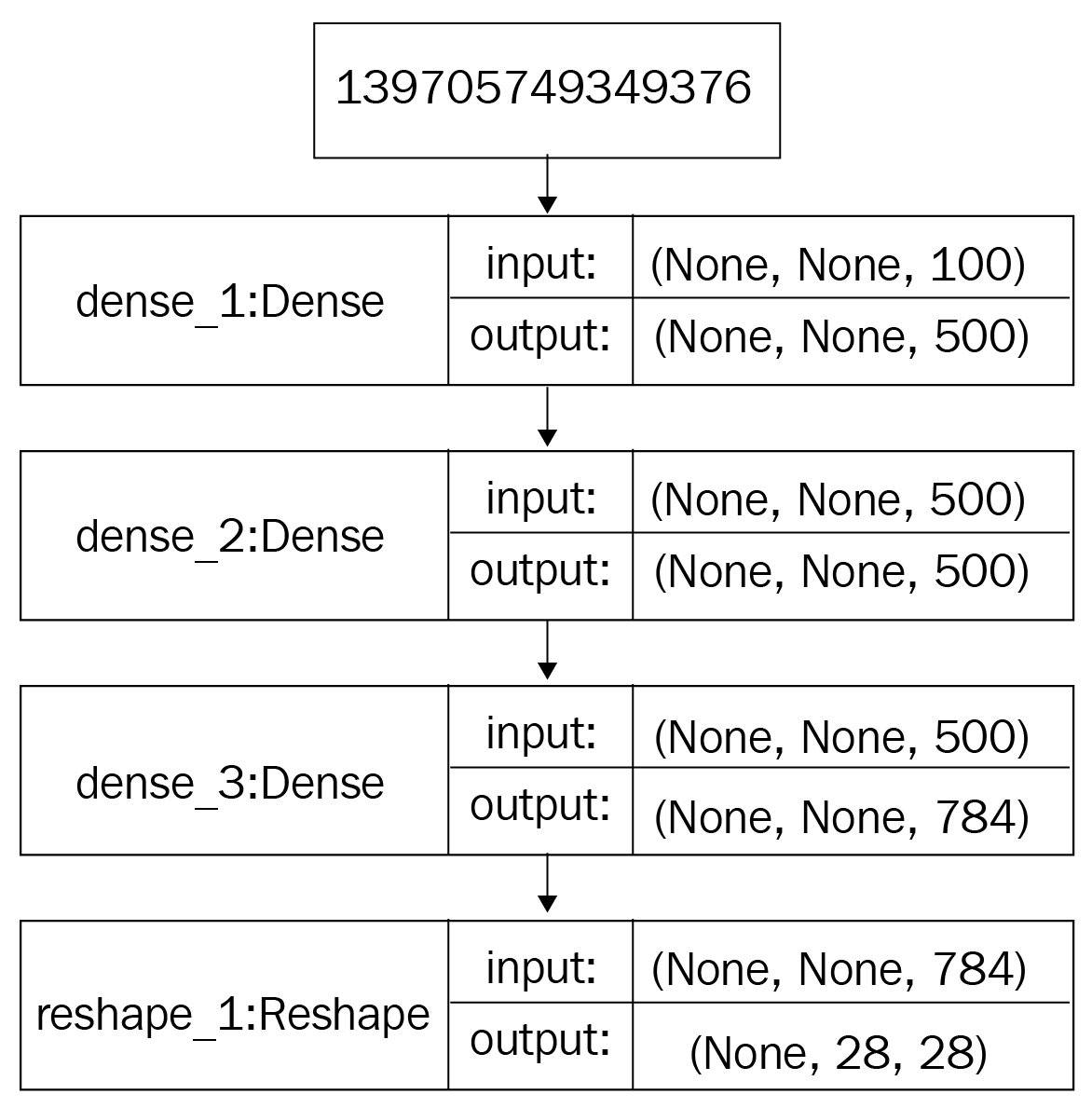The architecture of the generator network.

Let's discuss how this feed-forward neural network processes information during forward propagation of the data:

• The input layer takes aÂ 100-dimensional vector sampled from a Gaussian (normal) distributionÂ and passes the tensor to the first hidden layer without any modifications.
• The three hidden layers are dense layers with 500, 500, and 784 units, respectively.Â The first hidden layer (a dense layer) convertsÂ a tensor of a shape of (`batch_size, 100`) to a tensor of a shape of (`batch_size, 500`).
• The second dense layer generates a tensor of a shape ofÂ (`batch_size, 500`).
• The third hidden layer generates a tensor of a shape of (`batch_size, 784`).
• In the last output layer, this tensor is reshaped from a shape of (`batch_size, 784`) to a shape of (`batch_size, 28, 28`). This means that our network will generate a batch of images, where one image will have a shape of (28, 28).

### The architecture of the discriminator

The discriminator in our GAN is a feed-forward neural networkÂ with five layers, including an input and an output layer, and three dense layers.Â The discriminator network is a classifier and is slightly different from the generator network. It processes an image and outputs a probability of the image belonging to a particular class.

TheÂ following diagram showsÂ the flow of tensors and the input and output shapes of the tensors for each layer in the discriminator network: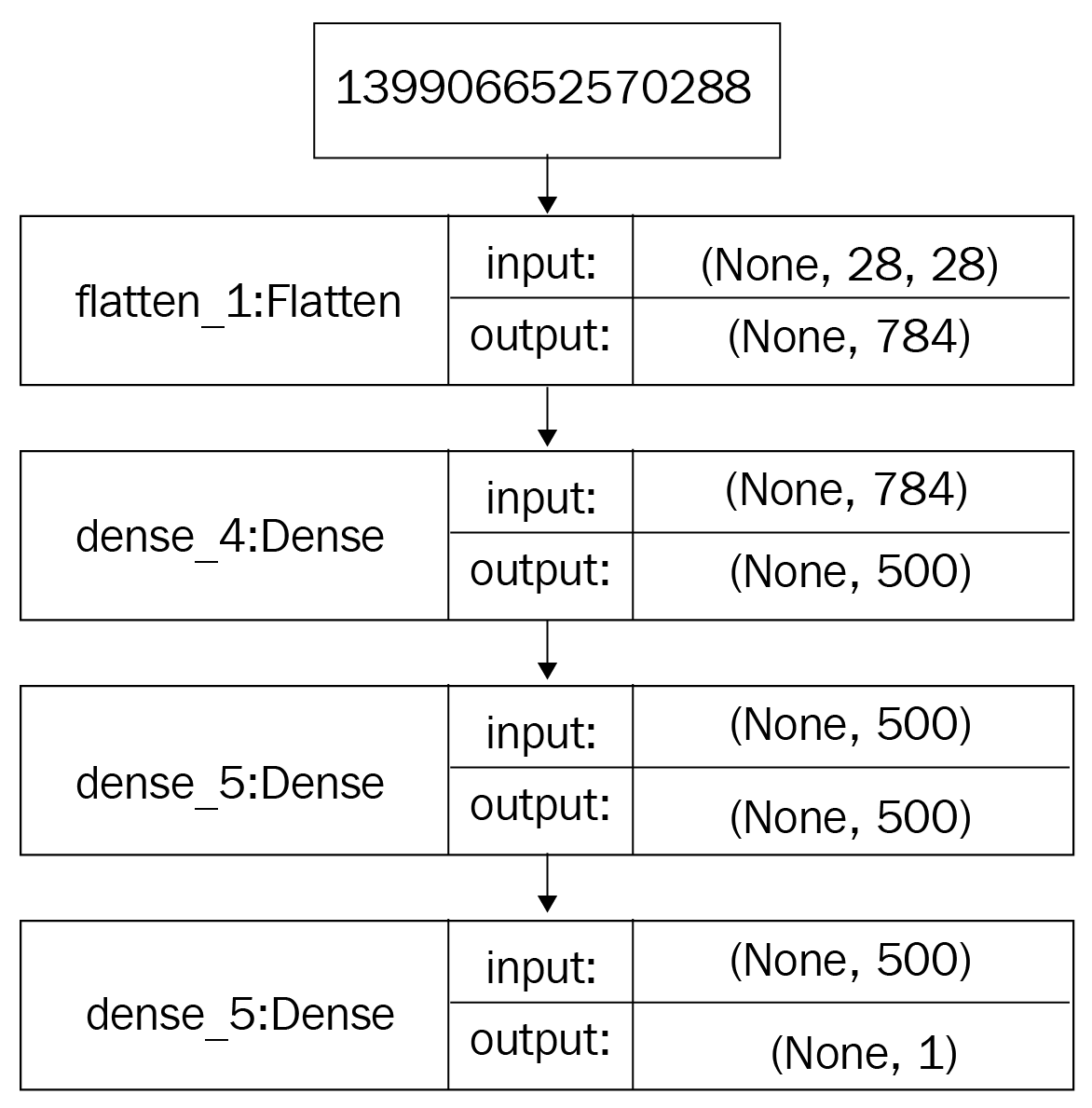The architecture of the discriminator network

Â

Let's discuss how the discriminator processes data in forward propagation during the training of the network:

1. Initially, itÂ receives an input of a shape of 28x28.Â
2. The input layer takes the input tensor, which is a tensor with a shape of (`batch_sizex28x28`), and passes it to the first hidden layer without any modifications.
3. Next, the flattening layer flattens the tensor toÂ a 784-dimensional vector, which gets passed to the first hidden (dense) layer. The first and second hidden layers modify this to a 500-dimensional vector.
4. The last layer is the output layer, which is again a dense layer, with one unit (aÂ neuron) and sigmoid as the activation function. It outputs a single value, either a 0 or a 1. A value of 0 indicates that the provided image is fake, while a value of 1 indicates that the provided image is real.

### Important concepts related to GANs

Now that we have understood the architecture of GANs, let's take a look at a brief overview of a few important concepts. We will first look atÂ KL divergence. It is very important to understand JS divergence, which is an important measure to assess the quality of the models. We will then look at the Nash equilibrium, which is a state that we try to achieve during training. Finally, we will look closer at objective functions, which are very important to understand in order to implement GANs well.

#### Kullback-Leibler divergence

Kullback-LeiblerÂ divergence (KL divergence), also known as relative entropy, is a method used to identify the similarity between two probability distributions. It measures how one probability distribution p diverges from a second expected probability distribution q.Â

The equation used to calculate the KL divergence between two probability distributions p(x) and q(x) is as follows:The KL divergence will be zero, or minimum, when p(x) is equal to q(x) at every other point.

Â

Â

Due to the asymmetric nature of KL divergence, we shouldn't use it to measure the distance between two probability distributions. It is therefore should not be used as a distance metric.

#### Jensen-Shannon divergence

The Jensen-ShannonÂ divergence (also called theÂ information radius (IRaD)Â or theÂ total divergence to the average) is another measure of similarity between two probability distributions. It is based on KL divergence.Â Unlike KL divergence, however, JS divergence is symmetric in nature and can be used to measure the distance between two probability distributions.Â If we take the square root of the Jensen-Shannon divergence, we get the Jensen-Shannon distance, so it is therefore a distance metric.

The followingÂ equation represents the Jensen-Shannon divergence between two probability distributions,Â p and q:In the preceding equation, (p+q) is the midpoint measure, whileÂÂ is the Kullback-Leibler divergence.

Now that we have learned about the KL divergence and the Jenson-Shannon divergence, let's discuss the Nash equilibrium for GANs.

#### Nash equilibrium

The Nash equilibrium describes a particular state in game theory. This state can be achieved in a non-cooperative game in which each player triesÂ to pick the best possible strategy to gain the best possible outcome for themselves, based on what they expect the other players to do. Eventually, all the players reach a point at which they have all picked the best possible strategy for themselves based on the decisions made by the other players. At this point in the game, they would gain no benefit from changing their strategy. This state is the Nash equilibrium.

A famous example of how the Nash equilibrium can be reached is with the Prisoner's Dilemma. In this example, two criminalsÂ (A and B)Â have been arrested for committing a crime. Both have been placed in separate cells with no way of communicating with each other. The prosecutor only has enough evidence to convict them for a smaller offense and not the principal crime, which would see them go to jail for a long time. To get a conviction, the prosecutor gives them an offer:

• If A and B both implicate each other in the principal crime, they both serve 2 years in jail.
• If A implicates B but B remains silent, A will be set free and B will serve 3 years in jail (and vice versa).
• If A and B both keep quiet, they both serve only 1 year in jail on the lesser charge.

From these three scenarios, it is obvious that the best possible outcome for A and B is to keep quiet and serve 1 year in jail. However, the risk of keeping quiet is 3 years as neither A nor B have any way of knowing that the other will also keep quiet. Thus, they would reach a state where their actual optimum strategy would be to confess as it is the choice that provides the highest reward and lowest penalty. When this state has been reached, neither criminal would gain any advantage by changing their strategy; thus, they would have reached a Nash equilibrium.

#### Objective functions

To create a generator network that generates images that are similar to real images, we try to increase the similarity ofÂ the data generated by the generator to real data. To measure the similarity, we use objective functions. Both networks have their own objective functions and during the training, they try toÂ minimize their respective objective functions.Â The following equation represents the final objective function for GANs: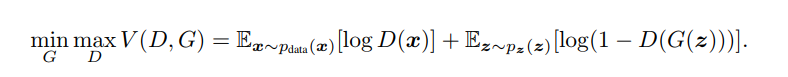In the preceding equation,Âis the discriminator model,Âis the generator model,Âis the real data distribution,Âis the distribution of the data generated by the generator, andÂ is the expected output.Â

During training, D (the Discriminator) wants to maximize the whole output and G (the Generator) wants to minimize it, thereby training a GAN to reach to an equilibrium between the generator and discriminator network. When it reaches an equilibrium, we say that the model has converged. This equilibrium is the Nash equilibrium.Â Once the trainingÂ is complete, we get a generator model that is capable of generating realistic-looking images.

### Scoring algorithms

Calculating the accuracy of a GAN is simple. The objective function for GANs is not a specific function, such as mean squared error or cross-entropy. GANs learn objective functions during training. There are many scoring algorithms proposed by researchers to measure how well a model fits. Let's look at some scoring algorithms in detail.

#### The inception score

The inception score is the most widely used scoring algorithm for GANs. It uses a pre-trained inception V3 network (trained on Imagenet) to extract the features of both generated and real images. It was proposed by Shane Barrat and Rishi Sharma in their paper,Â A Note on the Inception Score (https://arxiv.org/pdf/1801.01973.pdf). The inception score, or IS for short, measure the quality and the diversity of the generated images. Let's look at the equation for IS: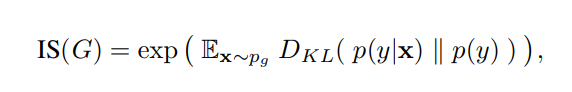In the preceding equation, notation x represents aÂ sample, sampled from a distribution.ÂandÂ represent the same concept.ÂÂ is the conditional class distribution, andÂÂ is the marginal class distribution.

To calculate the inception score, perform the following steps:

1. Start by sampling N number of images generated by the model, denoted as2. Then, construct the marginal class distribution, using the following equation:1. Then, calculate the KL divergence and the expected improvement, using the following equation:

Â1. Finally, calculate the exponential of the result to give us the inception score.

The quality of the model is good if it has a high inception score.Â Even though this is an important measure, it has certain problems. For example, it shows a good level of accuracy even when the model generates one image per class, which means the model lacks diversity. To resolve this problem, other performance measures were proposed. We will look at one of these in the following section.

To overcome the various shortcomings of the inception Score, the FrÃ©chlet Inception DistanceÂ (FID) was proposed by Martin Heusel and others in their paper,Â GANs Trained by a Two Time-Scale Update Rule Converge to a Local Nash Equilibrium (https://arxiv.org/pdf/1706.08500.pdf).Â

The equation to calculate the FID score is as follows:The preceding equation represents the FID score between the real images,Â x, and the generated images,Â g. To calculate the FID score, we use the Inception network to extract the feature maps from an intermediate layer in the Inception network. Then, we model a multivariate Gaussian distribution, which learns the distribution of the feature maps. This multivariate Gaussian distribution has a mean ofÂÂ and a covariance ofÂ, which we use to calculate the FID score. The lower the FID score, the better the model, and the more able it is to generate more diverse images with higher quality. A perfect generative model will have an FID score of zero. The advantage of using the FID score over the Inception score is that it is robust to noise and that it can easily measure the diversity of the images.

Â

### Note

The TensorFlow implementation of FID can be found at the following link:Â https://www.tensorflow.org/api_docs/python/tf/contrib/gan/eval/frechet_classifier_distance There are more scoring algorithms available that have been recently proposed by researchers in academia and industry. We won't be covering all of these here. Before reading any further, take a look at another scoring algorithm called the Mode Score, information about which can be found at the following link:Â https://arxiv.org/pdf/1612.02136.pdf.

## Variants of GANs

There are currently thousands of different GANs available and this number is increasing at a phenomenal rate. In this section, we will explore six popular GAN architectures, which we will cover in more detail in the subsequent chapters of this book.

### Deep convolutional generative adversarial networks

Alec Radford,Â Luke Metz, and Soumith Chintala proposed deep convolutional GANs (DCGANs) in a paper titled Unsupervised Representation Learning with Deep Convolutional Generative Adversarial Networks, which is available at the following link:Â https://arxiv.org/pdf/1511.06434.pdf. Vanilla GANs don't usually have convolutional neural networks (CNNs) in their networks. This was proposed for the first time with the introduction of DCGANs.Â We will learn how to generate anime character faces using DCGANs in Chapter 3,Â Face Aging Using Conditional GANs.

### StackGANs

StackGANs were proposed byÂ Han Zhang,Â Tao Xu,Â Hongsheng Li, and others in their paper titled StackGAN: Text to Photo-Realistic Image Synthesis with Stacked Generative Adversarial Networks, which is available at the following link:Â https://arxiv.org/pdf/1612.03242.pdf. They used StackGANs to explore text-to-image synthesis with impressive results. A StackGAN is a pair of networks that generate realistic looking images when provided with a text description. We will learn how to generate realistic looking images from text descriptions using a StackGAN in Chapter 6,Â StackGANÂ â€“ Text to Photo-Realistic Image Synthesis.

### CycleGANs

CycleGANs were proposed byÂ Jun-Yan Zhu,Â Taesung Park,Â Phillip Isola, andÂ Alexei A. Efros in a paper titled Unpaired Image-to-Image Translation using Cycle-Consistent Adversarial Networks, which is available at the following link:Â https://arxiv.org/pdf/1703.10593. CycleGANs have some really interesting potential uses, such as converting photos to paintings and vice versa, converting a picture taken in summer to a photo taken in winter and vice versa, or converting pictures of horses to pictures of zebras and vice versa. We will learn how to turn paintings into photos using a CycleGAN in Chapter 7,CycleGAN - Turn Paintings into Photos.

### 3D-GANs

3D-GANs were proposed byÂ Jiajun Wu,Â Chengkai Zhang,Â Tianfan Xue,Â William T. Freeman, andÂ Joshua B. Tenenbaum in their paper titled Learning a Probabilistic Latent Space of Object Shapes via 3D Generative-Adversarial Modeling, which is available at the following link:Â https://arxiv.org/pdf/1610.07584. Generating 3D models of objects has many use cases in manufacturing and the 3D modeling industry. A 3D-GAN network is able to generate new 3D models of different objects, once trained on 3D models of objects. We will learn how to generate 3D models of objects using a 3D-GAN in Chapter 2,Â 3D-GAN - Generating Shapes Using GAN.

### Age-cGANs

Face aging with Conditional GANs was proposed byÂ Grigory Antipov,Â Moez Baccouche, and Jean-Luc Dugelay in their paper titled Face Aging with Conditional Generative Adversarial Networks, which is available at the following link:Â https://arxiv.org/pdf/1702.01983.pdf. Face aging has many industry use cases, including cross-age face recognition, finding lost children, and in entertainment. We will learn how to train a conditional GAN to generate a face given a target age in Chapter 3,Â Face Aging Using Conditional GAN.

### pix2pix

The pix2pixÂ networkÂ was introduced byÂ Phillip Isola,Â Jun-Yan Zhu,Â Tinghui Zhou, andÂ Alexei A. Efros in their paper titled Image-to-Image Translation with Conditional Adversarial Networks, which is available at the following link:Â https://arxiv.org/abs/1611.07004. The pix2pix network has similar use cases to the CycleGAN network. It can convert building labels to pictures of buildingsÂ (we will see a similar exampleÂ in the pix2pix chapter), black and white images to color images, images taken in the day to night images, sketchesÂ to photos, and aerial images to map-like images.

### Note

For a list of all the GANs in existence, refer to The GAN Zoo, anÂ article by AvinashÂ Hindupur available atÂ https://github.com/hindupuravinash/the-gan-zoo.

GANs have certain advantages over other methods of supervised or unsupervised learning:

• GANs are an unsupervised learning method:Â Acquiring labeledÂ data is a manual process that takes a lot of time. GANs don't require labeledÂ data; they can be trained using unlabeledÂ data as they learn the internal representations of the data.
• GANs generate data:Â One of the best things about GANs is that they generate data that is similar to real data. Because of this, they have many different uses in the real world. They can generate images, text, audio, and video that is indistinguishable from real data. Images generated by GANs have applications in marketing, e-commerce, games, advertisements, and many other industries.

Â

Â

• GANs learn density distributions of data:Â GANsÂ learn theÂ internal representations of data. As mentioned earlier, GANs can learn messy and complicated distributions of data. This can be used for many machine learning problems.
• The trained discriminator is a classifier:Â After training, we get a discriminator and a generator. The discriminator network is a classifier and can be used to classify objects.

## Problems with training GANs

As with any technology, there are some problems associated with GANs. These problems are generally to do with the training process and include mode collapse, internal covariate shifts, and vanishing gradients. Let's look at these in more detail.

### Mode collapse

Mode collapse is a problem that refers to a situation in which the generator network generates samples that have little variety or when a model starts generating the same images. Sometimes, a probability distribution is multimodal and very complex in nature. This means that it might contain data from different observations and that it might have multiple peaks for different sub-graphs of samples. Sometimes, GANs fail to model a multimodal probability distribution of data and suffer from mode collapse. AÂ situation in which all the generated samples are virtually identical is known asÂ complete collapse.

There are many methods that we can use to overcome the mode collapse problem. These include the following:

• By training multiple models (GANs) for different modes

• By training GANs with diverse samples of data

During backpropagation, gradient flows backward, from the final layer to the first layer.Â As it flows backward, it gets increasingly smaller. Sometimes, the gradient is so small that the initial layers learn very slowly or stop learning completely.Â In this case, the gradient doesn't change the weight values of the initial layers at all, so the training of the initial layers in the network is effectively stopped. This is known as the vanishing gradients problem.

This problem gets worse if we train a bigger network with gradient-based optimization methods. Gradient-based optimization methods optimize a parameter's value by calculating the change in the network's output when we change the parameter's value by a small amount. IfÂ a change in the parameter's value causes a small change in the network's output, the weight change will be very small, so the network stops learning.

This is also a problem when we use activation functions, such as Sigmoid and Tanh. Sigmoid activation functions restrict values to a range of between 0 and 1, converting large values of x to approximately 1 and small or negative values of x to approximately zero.Â The Tanh activation function squashes input values to a range between -1 and 1, converting large input values to approximately 1 and small values to approximately minus 1. When we apply backpropagation, we use the chain rule of differentiation, which has a multiplying effect.As we reach the initial layers of the network, the gradient (the error) decreases exponentially,Â causing the vanishing gradients problem.

To overcome this problem, we can use activation functions such as ReLU, LeakyReLU, and PReLU. The gradients of these activation functions don't saturate during backpropagation, causing efficient training of neural networks. Another solution is to use batch normalization, which normalizes inputs to the hidden layers of the networks.

### Internal covariate shift

An internal covariate shift occurs when there is a change in the input distribution to our network. When the input distribution changes, hidden layers try to learn to adapt to the new distribution. This slows down the training process. If a process slows down, it takes a long time to converge to a global minimum. This problem occurs when the statistical distribution of the input to the networks is drastically different from the input that it has seen before.Â Batch normalization and other normalization techniques can solve this problem. We will explore these in the following sections.

## Solving stability problems when training GANs

Training stability is one of the biggest problems that occur concerning GANs. For some datasets, GANs never converge due to this type of problem. In this section, we will look at some solutions that we can use to improve the stability of GANs.

### Feature matching

During the training of GANs, we maximize the objective function of the discriminator network and minimize the objective function of the generator network. This objective function has some serious flaws. For example, it doesn't take into account the statistics of the generated data and the real data.

Feature matching is a technique that was proposed by Tim Salimans, Ian Goodfellow, and others in their paper titled Improved Techniques for Training GANs,Â to improve the convergence of the GANs by introducing a new objective function. The new objective function for the generator network encourages it to generate data, with statistics, that is similar to the real data.

To apply feature mapping, the network doesn't ask the discriminator to provide binary labels. Instead, the discriminator network provides activations or feature maps of the input data, extracted from an intermediate layer in the discriminator network. From a training perspective, we train the discriminator network to learn the important statistics of the real data; hence, the objective is that it should be capable of discriminating the real data from the fake data by learning those discriminative features.

To understand this approach mathematically, let's take a look at the different notations first:

•: The activation or feature maps for the real data from an intermediate layer in the discriminator network
•: The activation/feature maps for the data generated by the generator network fromÂ an intermediate layer in the discriminator network

This new objective function can be represented as follows: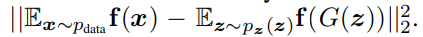Using this objective function can achieve better results, but there is still no guarantee of convergence.

Â

### Mini-batch discrimination

Mini-batch discrimination is another approach to stabilize the training of GANs. It was proposed by Ian Goodfellow and others in Improved Techniques for Training GANs, which is available atÂ https://arxiv.org/pdf/1606.03498.pdf. To understand this approach, let's first look in detail at the problem. While training GANs, when we pass the independent inputs to the discriminator network, the coordination between the gradients might be missing, and this prevents the discriminator network from learning how to differentiate between various images generated by the generator network. This is mode collapse, a problem we looked at earlier. To tackle this problem, we can use mini-batch discrimination. The following diagram illustrates the process very well: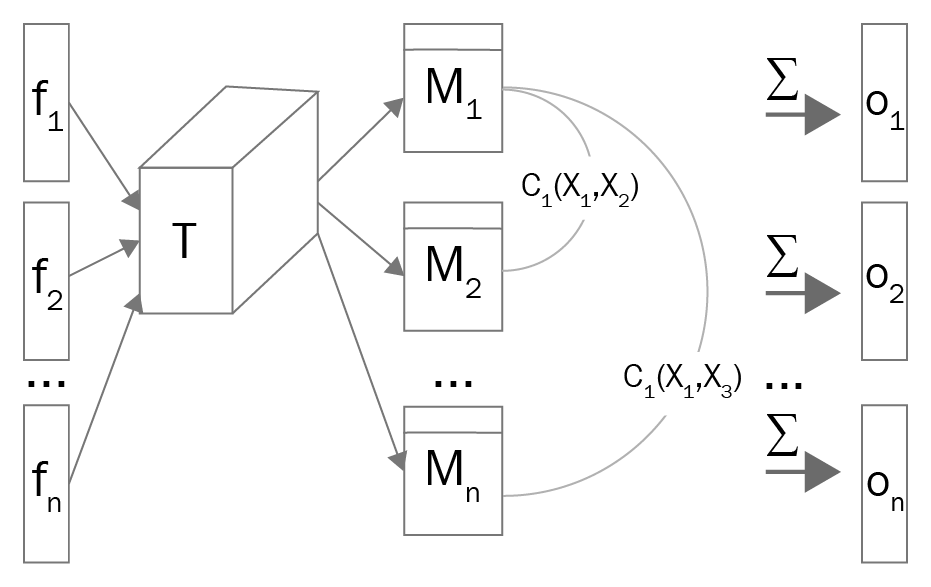Â

Â

Mini-batch discrimination is a multi-step process.Â Perform the following steps to add mini-batch discrimination to your network:

1. Extract the feature maps for the sample and multiply them by a tensor,Â, generating a matrix,Â.
2. Then, calculate the L1 distance between the rows of the matrixÂÂ using the following equation:1. Then, calculate the summation of all distances for a particular example,Â:1. Then, concatenateÂÂ withÂÂ  and feed it to the next layer of the network:To understand this approach mathematically, let's take a closer look at the various notions:

•:Â The activation or feature maps forÂÂ sample from an intermediate layer in the discriminator network
•: A three-dimensional tensor, which we multiply byÂ•: The matrix generated when we multiply the tensor T andÂ•: The output after taking the sum of all distances for a particular example,ÂMini-batch discrimination helps prevent mode collapse and improves the chances of training stability.

### Historical averaging

Historical averaging is an approach that takes the average of the parameters in the past and adds this to the respective cost functions of the generator and the discriminator network. It was proposed by Ian Goodfellow and others in a paper mentioned previously, Improved Techniques for Training GANs.

The historical average can be denoted as follows:In the preceding equation,ÂÂ is the value of parameters at a particular time, i. This approach can improve the training stability of GANs too.

### One-sided label smoothing

Earlier, label/target values for a classifier were 0 or 1; 0 for fake images and 1 for real images. Because of this, GANs were prone to adversarial examples, whichÂ are inputs to a neural network that result in an incorrect output from the network.Â Label smoothing is an approach to provide smoothed labels to the discriminator network. This means we can have decimal values such asÂ 0.9 (true), 0.8 (true), 0.1 (fake), or 0.2 (fake), instead of labeling every example as either 1 (true) or 0 (fake). We smooth the target values (label values) of the real images as well as of the fake images. Label smoothing can reduce the risk of adversarial examples in GANs. To apply label smoothing, assign the labels 0.9, 0.8, and 0.7, and 0.1, 0.2, and 0.3, to the images. To find out more about label smoothing, refer to the following paper:Â https://arxiv.org/pdf/1606.03498.pdf.

### Batch normalization

Batch normalization is a technique that normalizes the feature vectors to have no mean or unit variance. It is used to stabilize learning and to deal with poor weight initialization problems. It is a pre-processing step that we apply to the hidden layers of the network and it helps us to reduce internal covariate shift.

Batch normalization was introduced by Ioffe and Szegedy in their 2015 paper,Â Batch Normalization: Accelerating Deep Network Training by Reducing Internal Covariate Shift.Â This can be found at the following link:Â https://arxiv.org/pdf/1502.03167.pdf.

Â

The benefits of batch normalization are as follows:

• Reduces the internal covariate shift: Batch normalization helps us to reduce the internal covariate shift by normalizing values.
• Faster training: Networks will be trained fasterÂ if the valuesare sampled from a normal/Gaussian distribution. Batch normalization helps to whiten the values to the internal layers of our network. The overall training is faster, but each iteration slows down due to the fact that extra calculations are involved.
• Higher accuracy: Batch normalization provides better accuracy.
• Higher learning rate: Generally, when we train neural networks, we use a lower learning rate, which takes a long time to converge the network. With batch normalization, we can use higher learning rates, making our network reach the global minimum faster.
• Reduces the need for dropout:Â When we use dropout, we compromise some of the essential information in the internal layers of the network. Batch normalization acts as a regularizer, meaning we can train the network without a dropout layer.

In batch normalization, we apply normalization to all the hidden layers, rather than applying it only to the input layer.

### Instance normalization

As mentioned in the previous section, batch normalizationÂ normalizes a batch of samples by utilizing information from this batch only. Instance normalization is a slightly different approach. In instance normalization, we normalize each feature map by utilizing information from that feature map only. Instance normalization was introduced by Dmitry Ulyanov and Andrea Vedaldi in the paper titled Instance Normalization: The Missing Ingredient for Fast Stylization, which is available at the following link:Â https://arxiv.org/pdf/1607.08022.pdf.

Â

Â

## Summary

In this chapter, we learned about what a GAN is and which components constitute a standard GAN architecture. We also explored the various kinds of GANs that are available. After establishing the basic concepts of GANs, we moved on to looking at the underlying concepts that go into the construction and functioning of GANs. We learned about the advantages and disadvantages of GANs, as well as the solutions that help overcome those disadvantages. Finally, we learned about the various practical applications of GANs.

Using the fundamental knowledge of GANs in this chapter, we will now move on to the next chapter, where we will learn to generate various shapes using GANs.